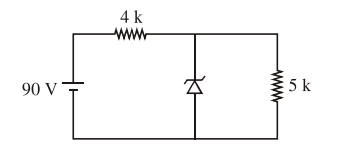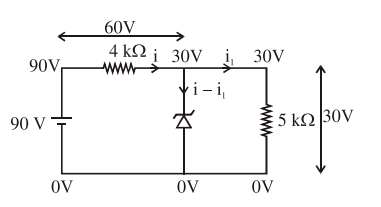Deepak Scored 45->99%ile with Bounce Back Crack Course. You can do it too!

# Solve this following

Question:

The zener diode has a $\mathrm{V}_{\mathrm{z}}=30 \mathrm{~V}$. The current passing through the diode for the following circuit is ......... mA.Solution:$i=\frac{60}{4000} A$

$\mathrm{i}_{1}=\frac{30}{5000} \mathrm{~A}$

$i-i_{1}=\frac{60}{4000}-\frac{30}{5000}=\frac{9}{1000} \mathrm{~A}$

current from zener diode

$\mathrm{i}_{\mathrm{z}}=\mathrm{i}-\mathrm{i}_{1}=9 \mathrm{~mA}$